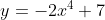Scan QR code or get instant email to install app

Question:

# 1212Which of the following best describes the range of?

A y ≤ 7
explanation

1212The range of a function describes the set of all outputs, y, that satisfy the equation defining the function. In the xy-plane, the graph ofis a U-shaped graph that opens downward with its vertex at (0, 7). Because the graph opens downward, the vertex indicates that the maximum value of y is 7. Therefore, the range of the function defined byis the set of y-values less than or equal to 7.